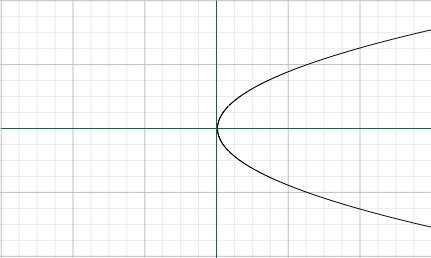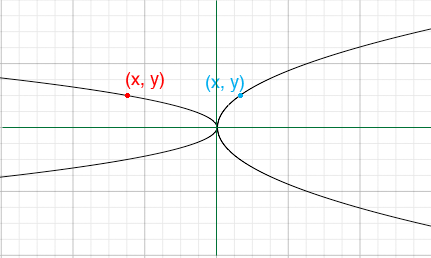SEARCH HOMEMath Central Quandaries & QueriesQuestion from Jil, a student: My question is when dealing with parabolas, x=y^2, so that they are shifted on their side you could say, what will happen to the graph if you change it to x=-4y^2. I understand that the - flips the graph in the other direction but can you simply just plug in numbers and increase the stretch of the y?Hi Jil,

As you indicated the parabola x = y2 is "on its side".x = y2

You can determine the shape of x = -4 y2 by substituting some numbers as you suggest. Sometimes you can see what happens without using specific points.

Suppose the curves are x = y2 and x = -4 y2 and and you want to find points on the two curves with the same y-value. Then substitute y2 from the first equation into the second to obtain

x = -4 x

So to achieve the same y-value the x-value on the second curve must be (minus) 4 times the x-value on the first curve.x = -4y2 and x = y2

I hope this helps,
PennyMath Central is supported by the University of Regina and The Pacific Institute for the Mathematical Sciences.